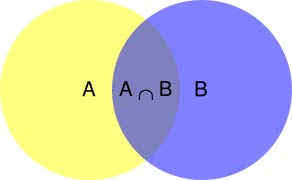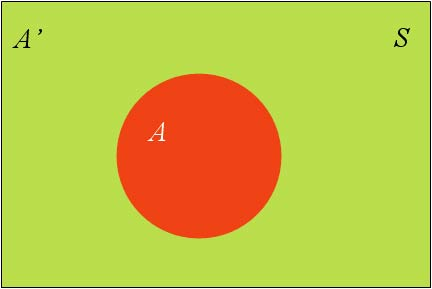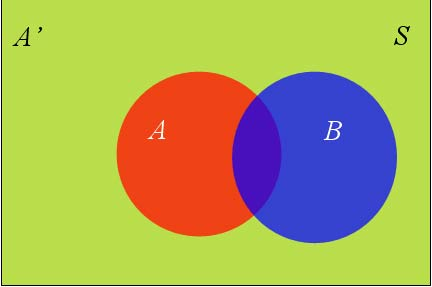# 性别推断

上官婉儿



# 开源工具

## maven 引入

<dependency>
<groupId>com.github.houbb</groupId>
<artifactId>chinese-name</artifactId>
<version>0.0.6</version>
</dependency>


## 使用

IChineseNameGenderProb genderProb = ChineseNameProbHelper.genderProb("上官婉儿");
Assert.assertEquals("ChineseNameGenderProb{gender=GIRL, prob=0.9780038580012211}", genderProb.toString());

IChineseNameGenderProb genderProb2 = ChineseNameProbHelper.genderProb("吴青峰");
Assert.assertEquals("ChineseNameGenderProb{gender=BOY, prob=0.8912627417339674}", genderProb2.toString());


# 什么是贝叶斯推断

ps: 这里也正是统计学的两大学派。贝叶斯学派和频率学派。

# 贝叶斯定理∵ P(A|B) = P(A∩B)/P(B)
∴ P(A∩B) = P(A|B)P(B)

P(A∩B) = P(B|A)P(A)
∴ P(A|B)P(B) = P(B|A)P(A)

P(A|B) = P(B|A)P(A)/P(B)


# 全概率公式P(B) = P(B∩A) +P(B∩A')
∵ P(B∩A) = P(B|A)P(A)
∴ P(B) = P(B|A)P(A) + P(B|A')P(A')


# 贝叶斯推断的含义

P(A|B) = P(A)·P(B|A)/P(B)


P(A|B) 称为”后验概率”（Posterior probability），即在B事件发生之后，我们对A事件概率的重新评估。

P(B|A)/P(B) 称为”可能性函数”（Likelyhood），这是一个调整因子，使得预估概率更接近真实概率。

后验概率　＝　先验概率 ｘ 调整因子


# 基于贝叶斯的性别推断

## 贝叶斯公式

 贝叶斯公式: P(Y X) = P(X Y) * P(Y) / P(X)
 当X条件独立时, P(X Y) = P(X1 Y) * P(X2 Y) * …

P(gender=男|name=青峰)
= P(name=青峰|gender=男) * P(gender=男) / P(name=青峰)
= P(name has 青|gender=男) * P(name has 山|gender=男) * P(gender=男) / P(name=青峰)


## 计算方式

•  怎么算 P(name has 青 gender=男) ?

“青”在男性名字中出现的次数 / 男性字出现的总次数

• 怎么算 P(gender=男)?

• 怎么算 P(name=青峰)?

## 数据准备

青,54716,48604



字，男性次数，女性次数


## 名字的概率计算

### 性别字典初始化

/**
* 男性字出现总数
* @author 老马啸西风
* @since 0.0.2
*/
private static long boyCharTotal;
/**
* 女性字出现总数
* @since 0.0.2
*/
private static long girlCharTotal;
/**
* 男性名字出现的概率
* @since 0.0.2
*/
private static double boyGenderProb;
/**
* 女性名字出现的概率
* @since 0.0.2
*/
private static double girlGenderProb;
/**
* count map
*
* @since 0.0.2
*/
private static Map<Character, Pair<Double, Double>> countMap;
static {
countMap = Guavas.newHashMap(lines.size());
List<ChineseNameGenderBean> beanList = Guavas.newArrayList(lines.size());
for(String line : lines) {
ChineseNameGenderBean bean = ChineseNameGenderBean.of(line);
boyCharTotal += bean.boyCount();
girlCharTotal += bean.girlCount();
}
// 频率计算
final double boyCharDouble = boyCharTotal*1.0;
final double girlCharDouble = girlCharTotal*1.0;
final double charTotalDouble = boyCharDouble + girlCharDouble;
boyGenderProb = boyCharDouble / charTotalDouble;
girlGenderProb = girlCharDouble / charTotalDouble;
// 频率在初始化的时候就计算好
for(ChineseNameGenderBean bean : beanList) {
double boyFreq = bean.boyCount() * 1.0 / boyCharDouble;
double girlFreq = bean.girlCount() * 1.0 / girlCharDouble;
Pair<Double, Double> pair = Pair.of(boyFreq, girlFreq);
countMap.put(bean.name(), pair);
}
}


（1）计算出 P(gender=性别) 的概率

 （2）计算出 P(name has 青 gender=男) 的概率。

### 概率的计算

java 实现如下：

//author: 老马啸西风
public double calcProb(GenderEnum genderEnum, String lastName) {
// 根据性别直接计算概率
double prob = getGenderProb(genderEnum);
// 遍历字对应的概率，还是要根据性别计算
// 如果为男性
char[] chars = lastName.toCharArray();
for(char c : chars) {
Pair<Double, Double> pair = countMap.get(c);
if(ObjectUtil.isNull(pair)) {
continue;
}
if(GenderEnum.BOY.equals(genderEnum)) {
prob *= pair.getValueOne();
} else {
// 女
prob *= pair.getValueTwo();
}
}
return prob;
}


getGenderProb 对应的是不同性别的概率，即 P(gender=男)/P(gender=女)。

/**
* 性别对应的概率
* @param genderEnum 性别枚举
* @return 概率结果
* @since 0.0.2
* @author 老马啸西风
*/
private double getGenderProb(final GenderEnum genderEnum) {
if(GenderEnum.BOY.equals(genderEnum)) {
return boyGenderProb;
}
return girlGenderProb;
}


public enum  GenderEnum {

BOY,

GIRL;
}


## 工具类封装

/**
* @author 老马啸西风
* @since 0.0.1
*/
public final class ChineseNameProbHelper {

private ChineseNameProbHelper(){}

/**
* 推断性别的概率
* @param fullName 全称
* @return 性别的概率推断
* @since 0.0.2
*/
public static IChineseNameGenderProb genderProb(final String fullName) {
String lastName = ChineseNameHelper.lastName(fullName);

return lastNameGenderProb(lastName);
}

/**
* 推断性别的概率
*
* @param lastName 名字
* @return 性别的概率推断
* @since 0.0.4
*/
public static IChineseNameGenderProb lastNameGenderProb(final String lastName) {
ChineseNameBs chineseNameBs = ChineseNameBs.newInstance();
return chineseNameBs.genderProb(lastName);
}

}


# 参考资料

NLP 中文人名生成器，性别识别实现思路